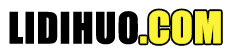# D3.js 缩放 API

D3.js 提供缩放功能来执行数据转换。这些函数将输入域映射到输出范围。

## 配置 API

```<script src = "https://d3js.org/d3-array.v1.min.js"></script>
<script src = "https://d3js.org/d3-collection.v1.min.js"></script>
<script src = "https://d3js.org/d3-color.v1.min.js"></script>
<script src = "https://d3js.org/d3-format.v1.min.js"></script>
<script src = "https://d3js.org/d3-interpolate.v1.min.js"></script>
<script src = "https://d3js.org/d3-time.v1.min.js"></script>
<script src = "https://d3js.org/d3-time-format.v2.min.js"></script>
<script src = "https://d3js.org/d3-scale.v1.min.js"></script>
<script>
</script>
```

## 缩放 API 方法

D3 为不同类型的图表提供了以下重要的缩放方法。让我们详细了解一下。
d3.scaleLinear()-构造一个连续的线性比例尺，我们可以在其中输入数据（域）映射到指定的输出范围。 d3.scaleIdentity()-构造一个线性比例，其中输入数据与输出相同。 d3.scaleTime()-构建一个线性比例尺，其中输入数据为日期，输出为数字。 d3.scaleLog()-构建对数刻度。 d3.scaleSqrt()-构造平方根刻度。 d3.scalePow()-构建指数尺度。 d3.scaleSequential()-构造一个序列标度，其中输出范围由插值器函数固定。 d3.scaleQuantize()-构造具有离散输出范围的量化标度。 d3.scaleQuantile()-构建一个分位数尺度，其中输入样本数据映射到离散输出范围。 d3.scaleThreshold()-构建一个比例尺，其中任意输入数据映射到离散输出范围。 d3.scaleBand()-波段尺度类似于序数尺度，但输出范围是连续的和数字的。 d3.scalePoint()-构建点比例尺。 d3.scaleOrdinal()-构造一个序数尺度，其中输入数据包括字母并映射到离散数字输出范围。

-域表示输入数据的最小值和最大值。 Range-Range 是输出范围，我们希望输入值映射到...

### 工作示例

```var data = [100, 200, 300, 400, 800, 0]
var width = 500,
barHeight = 20,
margin = 1;
```

```var scale = d3.scaleLinear()
.domain([d3.min(data), d3.max(data)])
.range([100, 400]);
```

```var svg = d3.select("body")
.append("svg")
.attr("width", width)
.attr("height", barHeight * data.length);
```

```var g = svg.selectAll("g")
.data(data).enter().append("g")
.attr("transform", function (d, i) {
return "translate(0," + i * barHeight + ")";
});
```

```g.append("rect")
.attr("width", function (d) {
return scale(d);
})
.attr("height", barHeight-margin)
```

```g.append("text")
.attr("x", function (d) { return (scale(d)); })
.attr("y", barHeight / 2)
.attr("dy", ".35em")
.text(function (d) { return d; });
```

```<!DOCTYPE html>
<html>
<script type = "text/javascript" src = "https://d3js.org/d3.v4.min.js"></script>
<body>
<script>
var data = [100, 200, 300, 350, 400, 250]
var width = 500, barHeight = 20, margin = 1;

var scale = d3.scaleLinear()
.domain([d3.min(data), d3.max(data)])
.range([100, 400]);

var svg = d3.select("body")
.append("svg")
.attr("width", width)
.attr("height", barHeight * data.length);

var g = svg.selectAll("g")
.data(data)
.enter()
.append("g")
.attr("transform", function (d, i) {
return "translate(0," + i * barHeight + ")";
});

g.append("rect")
.attr("width", function (d) {
return scale(d);
})

.attr("height", barHeight-margin)
g.append("text")
.attr("x", function (d) { return (scale(d)); })
.attr("y", barHeight / 2).attr("dy", ".35em")
.text(function (d) { return d; });
</script>
</body>
</html>
```